College Physics : Friction

Example Questions

Example Question #1 : Friction

Suppose a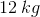box is being dragged across the floor due to a rope being pulled on at a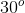angle from the side, as shown in the picture below.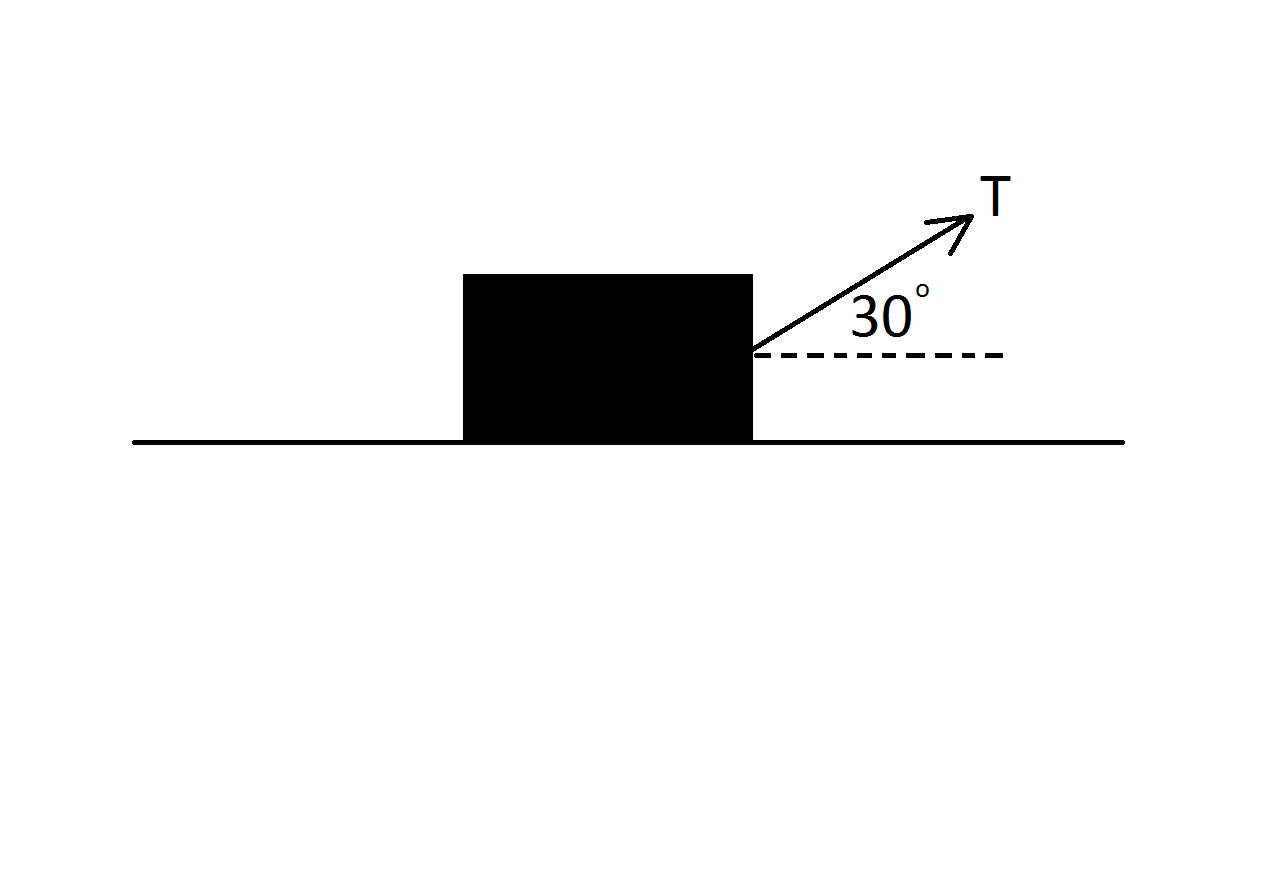If the tension in the rope is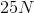and the box accelerates to the right at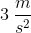, what is the coefficient of kinetic friction?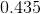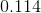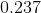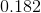Explanation:

To solve this problem, we first need to take into account the forces acting in the vertical direction separately from the forces acting in the horizontal direction.

First, let's start with the vertical direction. Here, the only force force acting downward is the weight of the box. There are two forces acting upwards on the box; one is the normal force and the other is the vertical component of the tension in the rope that is pulling the box. Because the box is not moving in the vertical direction, there is no net force. Thus, the sum of these forces is equal to zero.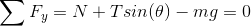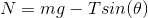Next, let's take a look at the forces acting horizontally on the box. Acting to the right of the box is the horizontal component of the tension in the rope. Acting to the left is the frictional force. Because the box is moving to the right, it must have experienced a net force in this direction. Thus, the sum of these horizontally acting forces will equal a net force.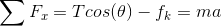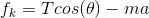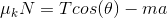Now, we can take the expression we obtained for the normal force and substitute it into the expression we obtained for the horizontally acting forces.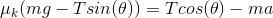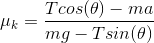Now that we've found an expression for the coefficient of kinetic friction, all we need to do is plug in the values given in the question stem to arrive at the answer.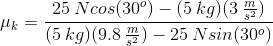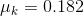All College Physics Resources